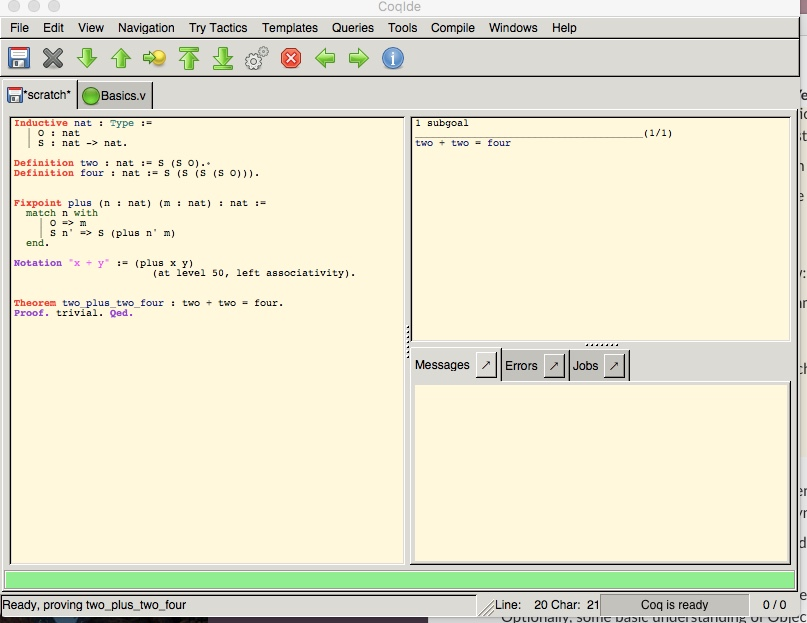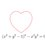# $2 + 2 = 4$ in Coq

Inspiration: Another Test Problem from Kelly's Group.

Coq is an interactive proof assistant. What this means is that Coq helps you prove theorems in a way that can be mechanically checked by Coq itself. This technology could be used to prove mathematical theorems, or develop formally correct software.

A famous example of a notorious theorem whose formal proof was developed in Coq is the four color theorem (download the proof here). Also, it has been used to generate a formally correct C compiler, which is pretty cool, if you think about it.

Behold we prove $2 + 2 = 4$ in Coq as a teaser

To run the proof below, you'll need Coq. You can read more about the Coq Proof Assistant on Wikipedia

We shall begin by defining the natural numbers along with two and four.

 1 2 3 4 5 6 Inductive nat : Type := | O : nat | S : nat -> nat. Definition two : nat := S (S O). Definition four : nat := S (S (S (S O))). 

We then, define plus

 1 2 3 4 5 6 7 8 Fixpoint plus (n : nat) (m : nat) : nat := match n with | O => m | S n' => S (plus n' m) end. Notation "x + y" := (plus x y) (at level 50, left associativity). 

Now, we can prove the theorem we desire.1 2 3 Theorem two_plus_two_four : two + two = four. Proof. simpl. reflexivity. Qed. 

And we are done!

Alternately, you could also use this:

 1 2 Theorem two_plus_two_four : two + two = four. Proof. trivial. Qed.4 years ago

This discussion board is a place to discuss our Daily Challenges and the math and science related to those challenges. Explanations are more than just a solution — they should explain the steps and thinking strategies that you used to obtain the solution. Comments should further the discussion of math and science.

When posting on Brilliant:

• Use the emojis to react to an explanation, whether you're congratulating a job well done , or just really confused .
• Ask specific questions about the challenge or the steps in somebody's explanation. Well-posed questions can add a lot to the discussion, but posting "I don't understand!" doesn't help anyone.
• Try to contribute something new to the discussion, whether it is an extension, generalization or other idea related to the challenge.

MarkdownAppears as
*italics* or _italics_ italics
**bold** or __bold__ bold
- bulleted- list
• bulleted
• list
1. numbered2. list
1. numbered
2. list
Note: you must add a full line of space before and after lists for them to show up correctly
paragraph 1paragraph 2

paragraph 1

paragraph 2

[example link](https://brilliant.org)example link
> This is a quote
This is a quote
    # I indented these lines
# 4 spaces, and now they show
# up as a code block.

print "hello world"
# I indented these lines
# 4 spaces, and now they show
# up as a code block.

print "hello world"
MathAppears as
Remember to wrap math in $$ ... $$ or $ ... $ to ensure proper formatting.
2 \times 3 $2 \times 3$
2^{34} $2^{34}$
a_{i-1} $a_{i-1}$
\frac{2}{3} $\frac{2}{3}$
\sqrt{2} $\sqrt{2}$
\sum_{i=1}^3 $\sum_{i=1}^3$
\sin \theta $\sin \theta$
\boxed{123} $\boxed{123}$

Sort by:

Another marvelous proof, you couldn't have eludicated any clearer!

I was thinking of proving 2+2=4 using accelerated course, but yours is definitely far superior to mine.

- 4 years ago